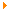• What is the 'Data Scan' option and how is it utilized?What is the 'Data Scan' option and how is it utilized?

The Data Scan feature is found under the Setup dialog list of options. This feature is designed for cases where the time channel data stream contains spurious "noise" like spikes (i.e. local maxima) that appear unrelated to the physical data measurement. The spikes are usually a single data point that generally exceeds the expected waveform profile. These spikes are sometimes observed when utilizing very low amplitude signals (i.e. approximately 1 millivolt full scale) in the presence of noisy ambient environments. The spikes can cause unwanted effects in post processing displays such as the time domain peak envelope processor found under the composite plot type option.

Setup and Operation
The data scan feature is configured on an individual channel basis. The user needs to supply a Trigger Reject Threshold (%) as well as a Multi-Pass Reject Count. A threshold of 0.0 will deactivate the scanner for the specified channel. The reject count indicates how many passes should be performed on each time domain data frame. Each pass can remove a single spurious "spike". Multiple passes are only necessary if multiple spikes are observed in the channel's displayed time domain waveform. The reject threshold specifies the rejection criterion based on an adjacent neighbor measurement. Once a spike has been identified, the neighbor data point values are examined to determine if the spike's value exceeds the threshold amount from the neighbor values. If the spike's value exceeds the specified threshold, then the spike value will be replaced with an arithmetic average of the neighbor data points.

Example - Consider the (time) data value sequence:

... 10., 15., 60., 15., 10., ..

The local spike is determined to be the value 60.0 (i.e. local maxima).If the threshold is specified as 100%, then the rejection criterion would be:

15. + 100% = 30.0

for both the left and right neighbor data values. Since the spike is 60.0, then that value would be replaced with the average value of:

(15.0 + 15.0)/2.0 = 15.0

Note that both neighbor points are utilized in the rejection criteria. Note also that only a single rejection is performed. If there is a possibility of multiple spikes in a data frame, then the user should specify a higher rejection count (greater than 1). One should generally keep the count low, such as <= 5 in order to not inadvertently alter the true character of the time domain waveform. If the rejection count is greater than 1; then once a spike has been corrected, a complete scan is performed to determine the next local maxima. The rejection processing is performed exactly the same as done for the first pass. If there were no rejected spikes during a scan, than all additional scans (if any are pending) will be skipped.